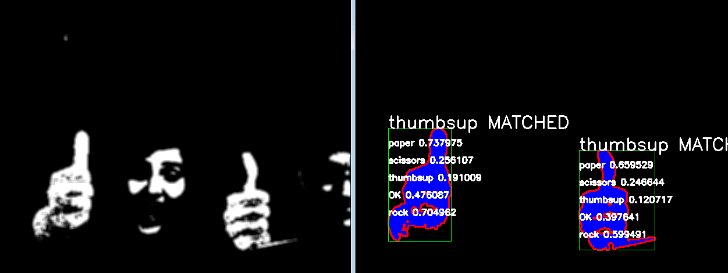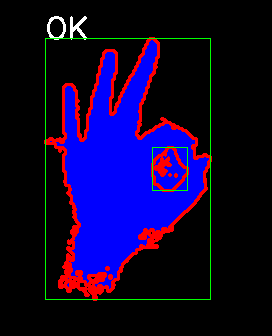Computer vision is easier than it seems thanks to libraries like OpenCV.

Here we will take a look at a previous assignment I worked on which implemented a rather rudimentary algorithm for detecting a group of hand signs.

First, let’s define what it means to recognize an object. In the context of this project, we’ll define it as successfully distinguishing hand signs from a given image or video feed and properly identifying the specific name of the hand sign if one exists.

This means that our program needs to complete essentially two main tasks:

1. Distinguish signs
2. Identify signs

Distinguishing objects from their surroundings is something we as humans do really well. When it comes to automating it, it seems rather confusing unless we know exactly what we are looking for. Luckily, we have a fairly good idea of what to look for! We know that a hand sign is composed of a hand which is an extension of a human being. It turns out that, despite vast ethnic differences, humans share a small range of skin colors which can be used to distinguish human parts from background images.To distinguish objects, we must apply a threshold function to essentially filter out everything that is not skin colored. We can do this by checking every pixel’s RGB value and creating a black & white image where white pixels are possible skin and black is background. Thanks to prior research, we also know what RGB values to look for:

• Red > 95
• Blue > 20
• Green > 40
• max(Red, Green, Blue) – min(Red, Green, Blue) > 15
• abs(Red – Green) > 15
• Red > Green
• Red > Blue

Once we have a thresholded image, we can then have OpenCV draw contours of the skin colored objects. Once the contours are drawn, we have successfully distinguished objects that could possibly be a body part such as a hand.

After that, we keep a limited number of only the biggest contours and discard the rest. Since we are repeating all of these processes multiple times in less than a second, we need to be conscious about our system resources and execution time. Considering only the biggest contours allows us to investigate objects that are most likely to be hand signs as they tend to be closer to the camera than background objects which means they will have bigger contours.

Each remaining contour then gets compared to every single pre-computed hand sign contours in our system. Before we start processing images or a video feed, we must process sample images for hand signs we’d like to recognize. You can see processed images for some of the hand signs used in our system.The comparison is done by utilizing the matchShapes function of OpenCV. The result is a number that represents the dissimilarity of two contours, i.e. closer to zero the more similar two contours are. In our case, we experimentally determined acceptable dissimilarity values which yielded reliable results. There are also very complex theoretical methods to do this.

Different hand signs are inherently similar to each other which needs to be alleviated as you could get false positives. (e.g. identifying a thumbs up while it in fact is a high five..) This can be done by picking the least dissimilar identification that is also below its predetermined acceptable value.

Here is an example code with in-line comments. The procedures outlined above are walked through in the code step by step.

```// Necessary libraries
#include "stdafx.h"
#include "opencv2/core/core.hpp"
#include "opencv2/highgui/highgui.hpp"
#include "opencv2/imgproc/imgproc.hpp"
#include <iostream>
#include <stdio.h>
#include <stdlib.h>
#include <numeric>
#include <vector>

// Set up namespaces so we don't bother with them later
using namespace cv;
using namespace std;

const int numGestures = 5;	//Total number of gestures
int maxComparison = 4;	//Maximum number of contours to compare. If more contours exist, only the biggest will be compared. Saves time.
double gestureThresholds[numGestures] = { 0.2, 0.25, 0.25, 0.21,  .2};	//Dissimilarity constants for each gesture obtained experimentally
string gestureNames[numGestures] = { "paper", "scissors", "thumbsup", "OK", "rock" };	//Names of our gestures

//Boring stuff.....
int myMax(int a, int b, int c);
int myMin(int a, int b, int c);
void mySkinDetect(Mat& src, Mat& dst);
void contourize(Mat src, vector<vector<Point>>& contours, vector<Vec4i>& hierarchy, int& maxsize, int& maxind);

//This is where everything happens
int main()
{
Mat src; Mat src_gray; Mat blur_gray;

//Start up a bunch of windows to display different states of our image processing operation
namedWindow("frame", WINDOW_AUTOSIZE);
namedWindow("skins", WINDOW_AUTOSIZE);
namedWindow("Contours", CV_WINDOW_AUTOSIZE);
namedWindow("gesture0", CV_WINDOW_AUTOSIZE);
namedWindow("gesture1", CV_WINDOW_AUTOSIZE);
namedWindow("gesture2", CV_WINDOW_AUTOSIZE);
namedWindow("gesture3", CV_WINDOW_AUTOSIZE);

//Vectors for storing the information processed from the images and contours on the go
vector<Mat> gestures(numGestures);
vector<vector<vector<Point>>> gesturesContours(numGestures);
vector<vector<Vec4i>> gesturesHierarchy(numGestures);
vector<int> gesturesMaxsize(numGestures);
vector<int> gesturesMaxind(numGestures);
vector<Mat> gesturesOutput(numGestures);
vector<Rect> gesturesBoundrec(numGestures);

//Create contours for our sample gesture/handsign images
for (int i = 0; i < numGestures; i++){
if (i == 0){ address = "paper.png"; }
else if (i == 1){ address = "scissors.png"; }
else if (i == 2){ address = "thumbsup.png"; }
else if (i == 3){ address = "osign.png"; }
else if (i == 4){ address = "rock.png"; }
else{ break; }

cout << "1" << endl;
blur(gestures[i], gestures[i], Size(3, 3));
cout << "2" << endl;
cvtColor(gestures[i], gestures[i], CV_BGR2GRAY);

cout << "3" << endl;
gesturesOutput[i] = Mat::zeros(gestures[i].size(), CV_8UC3);
cout << "4" << endl;
contourize(gestures[i], gesturesContours[i], gesturesHierarchy[i], gesturesMaxsize[i], gesturesMaxind[i]);
drawContours(gesturesOutput[i], gesturesContours[i], gesturesMaxind[i], Scalar(255, 0, 0), CV_FILLED, 8, gesturesHierarchy[i]);
drawContours(gesturesOutput[i], gesturesContours[i], gesturesMaxind[i], Scalar(0, 0, 255), 2, 8, gesturesHierarchy[i]);
gesturesBoundrec[i] = boundingRect(gesturesContours[i][gesturesMaxind[i]]);
rectangle(gesturesOutput[i], gesturesBoundrec[i], Scalar(0, 255, 0), 1, 8, 0);

}

//Render the sample images
imshow("gesture0", gesturesOutput);
imshow("gesture1", gesturesOutput);
imshow("gesture2", gesturesOutput);
imshow("gesture3", gesturesOutput);

//Acquire video feed
VideoCapture cap(0);
if (!cap.isOpened())
{
cout << "Cannot open the video cam" << endl;
return -1;
}
Mat frame0;
if (!bSuccess0)
{
cout << "Cannot read a frame from video stream" << endl;
}

//Keep processing images continously
while (1){
Mat frame;
//Check to see if we were able to read-in an image
if (!bSuccess)
{
cout << "Cannot read a frame from video stream" << endl;
break;
}
imshow("frame", frame);

//Try to detect skin colors
Mat skins;
mySkinDetect(frame, skins);
blur(skins, skins, Size(6, 6));
imshow("skins", skins);

Mat processed;
processed = skins.clone();

//Find contours in the skin color image
vector<vector<Point>> contours;
vector<Vec4i> hierarchy;
findContours(processed, contours, hierarchy, CV_RETR_TREE, CV_CHAIN_APPROX_NONE, Point(0, 0));
cout << "The number of contours detected is: " << contours.size() << endl;

//Calculate invariants for reference
vector<Moments> mu(contours.size());
for (int i = 0; i < contours.size(); i++)
{
mu[i] = moments(contours[i], false);
}
vector<Point2f> mc(contours.size());
for (int i = 0; i < contours.size(); i++)
{
mc[i] = Point2f(mu[i].m10 / mu[i].m00, mu[i].m01 / mu[i].m00);
}

Mat contour_output = Mat::zeros(processed.size(), CV_8UC3);

int maxsize = 0;
int maxind = 0;
Rect boundrec;

maxComparison = min(maxComparison, int(contours.size()));
vector<int> maxinds;
vector<int> maxsizes;
vector<Rect> boundrecs;

//Find the biggest contours and keep them
for (int i = 0; i < maxComparison; i++){
if (i == 0){
for (int j = 0; j < contours.size(); j++)
{
// Documentation on contourArea: http://docs.opencv.org/modules/imgproc/doc/structural_analysis_and_shape_descriptors.html#
double area = contourArea(contours[j]);
if (area > maxsize) {
maxsize = area;
maxind = j;
boundrec = boundingRect(contours[j]);
}
}
maxinds.push_back(maxind);
maxsizes.push_back(maxsize);
boundrecs.push_back(boundrec);
maxsize = 0;
maxind = 0;
}
else{
for (int j = 0; j < contours.size(); j++)
{
if (!(find(maxinds.begin(), maxinds.end(), j) != maxinds.end())){
double area = contourArea(contours[j]);
if (area > maxsize) {
maxsize = area;
maxind = j;
boundrec = boundingRect(contours[j]);
}
}
}
maxinds.push_back(maxind);
maxsizes.push_back(maxsize);
boundrecs.push_back(boundrec);
}
}

cout << "-----------------------------" << endl << endl;

/// Show in a window

double gestureValues[numGestures];
fill_n(gestureValues, numGestures, 100);
int gestureMatch[numGestures];

//Draw Contours
for (int i = 0; i < maxComparison; i++){
drawContours(contour_output, contours, maxinds[i], Scalar(255, 0, 0), CV_FILLED, 8, hierarchy);
drawContours(contour_output, contours, maxinds[i], Scalar(0, 0, 255), 2, 8, hierarchy);
rectangle(contour_output, boundrecs[i], Scalar(0, 255, 0), 1, 8, 0);
}

//Calculate similarity and draw on screen
for (int j = 0; j < maxComparison; j++){
for (int i = 0; i < numGestures; i++){
float ret = matchShapes(gesturesContours[i][gesturesMaxind[i]], contours[maxinds[j]], 1, 0.0);

string text;
text = gestureNames[i] + " " + to_string(ret);
int fontFace = FONT_HERSHEY_SIMPLEX;
double fontScale = 0.5;
int thickness = 2;
int baseline = 0;
Size textSize = getTextSize(text, fontFace, fontScale, thickness, &baseline);
baseline += thickness;
Point textOrg(boundrecs[j].x, boundrecs[j].y + 30 * (i + 1));
putText(contour_output, text, textOrg, fontFace, fontScale, Scalar::all(255), thickness, 8);

if (ret < gestureValues[i]){
gestureValues[i] = ret;
gestureMatch[i] = j;
}
}
double lowestgesture = 100;
int lowestgestureID = -1;
for(int i = 0; i < numGestures; i++){
if (lowestgesture >= gestureValues[i] && gestureValues[i] < gestureThresholds[i]){
lowestgesture = gestureValues[i];
lowestgestureID = i;
}
}
if (lowestgestureID != -1){
cout << "Match Found For " << gestureNames[lowestgestureID] << endl;
string text = gestureNames[lowestgestureID] + " MATCHED";
int fontFace = FONT_HERSHEY_SIMPLEX;
double fontScale = 1;
int thickness = 2;
int baseline = 0;
Size textSize = getTextSize(text, fontFace, fontScale, thickness, &baseline);
baseline += thickness;
Point textOrg(boundrecs[gestureMatch[lowestgestureID]].x, boundrecs[gestureMatch[lowestgestureID]].y);
putText(contour_output, text, textOrg, fontFace, fontScale, Scalar::all(255), thickness, 8);
}
}

//Display the resultant plot
imshow("Contours", contour_output);

//Exit when ESC key is pressed
if (waitKey(30) == 27)
{
cout << "Manual Exit" << endl;
break;
}
}

//Release camera
cap.release();
return(0);
}

//Function that returns the maximum of 3 integers
int myMax(int a, int b, int c) {
return max(max(a, b), c);
}

//Function that returns the minimum of 3 integers
int myMin(int a, int b, int c) {
return min(min(a, b), c);
}

void mySkinDetect(Mat& src, Mat& dst) {
//Thresholding function to filter out background and set skin colored pixels to white
cvtColor(src, dst, CV_BGR2GRAY);

for (int i = 0; i < src.rows; i++)
{
for (int j = 0; j < src.cols; j++)
{
int pBlue = src.at<cv::Vec3b>(i, j);
int pGreen = src.at<cv::Vec3b>(i, j);
int pRed = src.at<cv::Vec3b>(i, j);
if (pRed > 95 && pBlue > 20 && pGreen > 40 && myMax(pRed, pGreen, pBlue) - myMin(pRed, pGreen, pBlue) > 15 && abs(pRed - pGreen) > 15 && pRed > pGreen && pRed > pBlue)
{
dst.at<uchar>(i, j) = 255;
}
else
{
dst.at<uchar>(i, j) = 0;
}
}
}

}

void contourize(Mat src, vector<vector<Point>>& contours, vector<Vec4i>& hierarchy, int& maxsize, int& maxind){
//Draws contours around individual skin-colored objects
findContours(src, contours, hierarchy, CV_RETR_TREE, CV_CHAIN_APPROX_SIMPLE, Point(0, 0));

//Rect boundrec;
for (int i = 0; i < contours.size(); i++)
{
// Documentation on contourArea: http://docs.opencv.org/modules/imgproc/doc/structural_analysis_and_shape_descriptors.html#
double area = contourArea(contours[i]);
if (area > maxsize) {
maxsize = area;
maxind = i;
}
}
}```

Vezhnevets, Vladimir, Vassili Sazonov, and Alla Andreeva. “A survey on pixel-based skin color detection techniques.” Proc. Graphicon. Vol. 3. 2003.

Kakumanu, Praveen, Sokratis Makrogiannis, and Nikolaos Bourbakis. “A survey of skin-color modeling and detection methods.” Pattern recognition 40.3 (2007): 1106-1122.

Special thanks to Professor Margrit Betke, Ajjen Joshi and my teammates Maria Kromis and Abesary Woldeyesus.